﻿ LES和DNS在平坡异重流数值模拟中的比较研究

# LES和DNS在平坡异重流数值模拟中的比较研究Comparative Study of LES and DNS on Numerical Simulation of Gravity Current over a Flat Bed

Abstract: In this paper, the FLUENT software was used to simulate the evolution characteristics of the lock-exchange gravity currents over a flat bed. Two-dimensional large eddy simulation (LES) and direct numerical simulation (DNS) were utilized to compare the morphological change, instantane-ous mixing coefficient, potential energy transformation, and kinetic energy at the characteristic section. The results show that both LES and DNS have good accuracy in predicting the head position of the gravity currents, but the results of DNS are slightly more accurate with more computational grids required. For simulating the development process of gravity currents, the DNS results show less K-H instability occurring at the interface of current and ambient fluid than the LES simulations do. For DNS simulations, heavy fluid basically concentrates on the current head. In addition, the DNS results are closer to the gravity current morphology from experimental observations. The mixing degree of density flow with ambient fluid estimated by LES and DNS simulations is similar, but the result of DNS shows smoother current-ambient fluid interface. LES and DNS results obtain similar potential energy changes along the course of density flow, but the results of DNS have smaller background potential energy. For the temporal variations of kinetic energy at characteristic cross-sections, compared with LES, DNS performs fewer oscillating peaks. The results of this paper can provide a reference for the selection of numerical models on gravity current simulations in the future.

1. 引言

2. 数值模型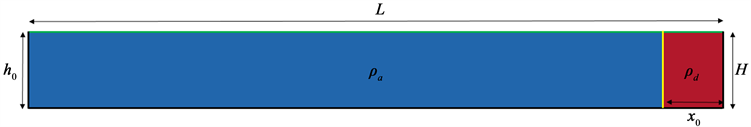Figure 1. Schematic diagram of physical model

2.1. 数值模型

2.1.1. 网格及求解方法

2.1.2. 边界条件

2.2. 模型验证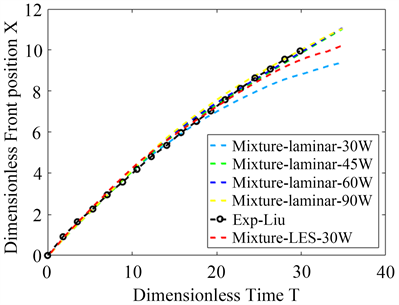Figure 2. Comparison of temporal head position of gravity current between numerical and experimental results

$X=\frac{{x}_{f}-{x}_{0}}{H}$ (1)

$T=\frac{t\sqrt{{g}^{\prime }H}}{H}$ (2)

2.3. 特征参数及组别Table 1. Parameters of numerical cases

3. 结果分析

3.1. 流态分析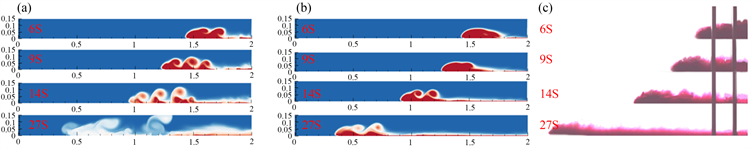Figure 3. Development processes of gravity current in the case N3: (a) LES; (b) DNS; (c) Experimental results

3.2. 卷吸和掺混

${E}_{i}=\frac{2\left({M}_{i}-{M}_{i-1}\right)}{\left({x}_{f,i}+{x}_{f,i-1}\right)\left({U}_{b,i}+{U}_{b,i-1}\right)\left({t}_{i}-{t}_{i-1}\right)}$ (3)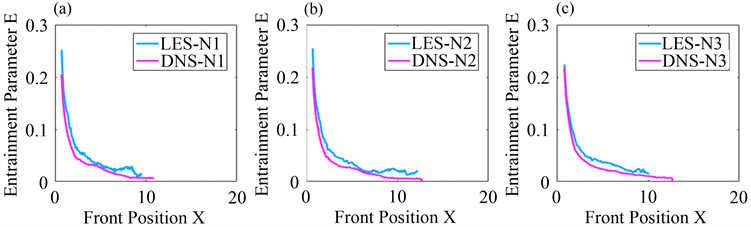Figure 4. The variation of entrainment parameter with front position of gravity current: (a) N1; (b) N2; (c) N3

3.3. 势能转换

${E}_{p}\left(t\right)=g\underset{V}{\int }〈\rho \left(x,z,t\right)〉z\text{d}V$ (4)

${E}_{b}\left(t\right)=g\underset{V}{\int }〈\stackrel{˜}{\rho }\left(x,z,t\right)〉z\text{d}V$ (5)

${E}_{a}\left(t\right)={E}_{p}\left(t\right)-{E}_{b}\left(t\right)$ (6)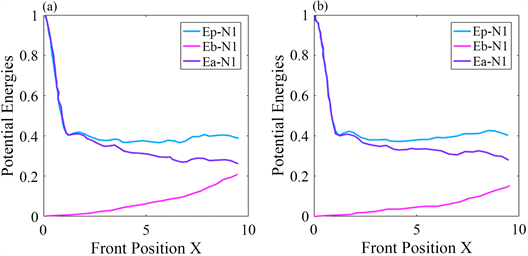Figure 5. The variation of the dimensionless total potential energy, background potential energy, and available potential energy with head position of gravity current in N1: (a) LES; (b) DNS

3.4. 动能峰值

$K=\frac{1}{2}\underset{\Omega }{\int }{\stackrel{¯}{u}}^{2}+{\stackrel{¯}{w}}^{2}\text{d}\Omega$ (7)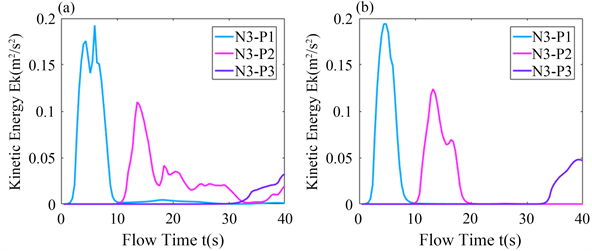Figure 6. Kinetic energy profile at the characteristic section of density currents of the case N3: (a) LES; (b) DNS

4. 结语

1) LES和DNS对于异重流头部位置的预测都具有较好的准确性，DNS的结果略微精确，但所需网格数较多；

2) 在异重流与环境流体的交界面发展过程中，相比于LES模拟的结果，DNS的结果具有较少的K-H不稳定性产生，而DNS模拟的结果与实验异重流的形态较为接近；

3) LES和DNS模拟计算得出的异重流与环境流体的掺混程度相近，但DNS的交界面较为光滑；

4) LES和DNS对于异重流沿途势能变化的计算结果相近，但DNS的结果具有较小的背景势能；

5) 对于特征断面处动能逐时变化，相较于LES，DNS模拟较少出现多次波峰的震荡现象。

NOTES

*通讯作者。

 Simpson, J.E. (1982) Gravity Currents in the Laboratory, Atmosphere, and Ocean. Annual Review of Fluid Mechanics, 14, 213-234.
https://doi.org/10.1146/annurev.fl.14.010182.001241

 高亚军, 陆永军, 李国斌. 黄河小浪底库区高含沙异重流的运动过程分析[J]. 水利水运工程学报, 2008(2): 60-66.

 张翔, 邱秀云, 李琳, 赵涛, 罗菲. 垂向异重流式分离鳃在静水中的集成试验[J]. 水利水运工程学报, 2011(2): 89-93.

 Ottolenghi, L., Ad-duce, C., Inghilesi, R., et al. (2016) Mixing in Lock-Release Gravity Currents Propagating up a Slope. Physics of Fluids, 28, 056604.
https://doi.org/10.1063/1.4948760

 Ottolenghi, L., Adduce, C., Inghilesi, R., et al. (2016) En-trainment and Mixing in Unsteady Gravity Currents. Journal of Hydraulic Research, 54, 541-557.
https://doi.org/10.1080/00221686.2016.1174961

 Steenhauer, K., Tokyay, T. and Constantinescu, G. (2017) Dynamics and Structure of Planar Gravity Currents Propagating Down an Inclined Surface. Physics of Fluids, 29, 036604.
https://doi.org/10.1063/1.4979063

 Meiburg, E., Radhakrishnan, S. and Nasr-Azadani, M. (2015) Modeling Gravity and Turbidity Currents: Computational Approaches and Challenges. Applied Mechanics Reviews, 67, 040802.
https://doi.org/10.1115/1.4031040

 Necker, F., Hartel, C., Kleiser, L. and Meiburg, E. (2002) High-Resolution Simulations of Particle-Driven Gravity Currents. International Journal of Multiphase Flow, 28, 279-300.
https://doi.org/10.1016/S0301-9322(01)00065-9

 Ooi, S.K., Constantinescu, G. and Weber, L.J. (2007) 2D Large-Eddy Simulation of Lock-Exchange Gravity Current Flows at High Grashof Numbers. Journal of Hy-draulic Engineering, 133, 1037-1047.
https://doi.org/10.1061/(ASCE)0733-9429(2007)133:9(1037)

 Nourazar, S. and Safavi, M. (2017) Two-Dimensional Large-Eddy Simulation of Density-Current Flow Propagating up a Slope. Journal of Hydraulic Engi-neering, 143, 4017035.
https://doi.org/10.1061/(ASCE)HY.1943-7900.0001329

 Härtel, C., Carlsson, F. and Thunblom, M. (2000) Analysis and Direct Numerical Simulation of the Flow at a Gravity-Current Head. Part 2. The Lobe-and-Cleft Instability. Journal of Fluid Mechanics, 418, 213-229.
https://doi.org/10.1017/S0022112000001270

 Härtel, C., Meiburg, E. and Necker, F. (2000) Analysis and Di-rect Numerical Simulation of the Flow at a Gravity-Current Head. Part 1. Flow Topology and Front Speed for Slip and No-Slip Boundaries. Journal of Fluid Mechanics, 418, 189-212.
https://doi.org/10.1017/S0022112000001221

 Birman, V.K., Martin, J.E. and Meiburg, E. (2005) The Non-Boussinesq Lock-Exchange Problem. Part 2. High-Resolution Simulations. Journal of Fluid Mechanics, 537, 125-144.
https://doi.org/10.1017/S0022112005005033

 Liu, X. and Jiang, Y. (2014) Direct Numerical Simula-tions of Boundary Condition Effects on the Propagation of Density Current in Wall-Bounded and Open Channels. Envi-ronmental Fluid Mechanics, 14, 387-407.
https://doi.org/10.1007/s10652-013-9283-6

 林颖典, 刘雅钰, 袁野平. 在层结和非层结环境下植被群对异重流运动的影响[J]. 吉林大学学报(地球科学版), 2019, 49(6): 1714-1722.

 Winters, K.B., Lombard, P.N., Riley, J.J. and D’Asaro, E.A. (1995) Available Potential Energy and Mixing in Density-Stratified Fluids. Journal of Fluid Me-chanics, 289, 115-128.
https://doi.org/10.1017/S002211209500125X

 Li, C.W. and Zhang, M.L. (2010) Nu-merical Modeling of Shallow Water Flow around Arrays of Emerged Cylinders. Journal of Hydro-Environment Re-search, 4, 115-121.
https://doi.org/10.1016/j.jher.2010.04.005

 Kneller, B. and Buckee, C. (2000) The Struc-ture and Fluid Mechanics of Turbidity Current: A Review of Some Recent Studies and Their Geological Implications. Sedimentology, 47, 62-94.
https://doi.org/10.1046/j.1365-3091.2000.047s1062.x

Top